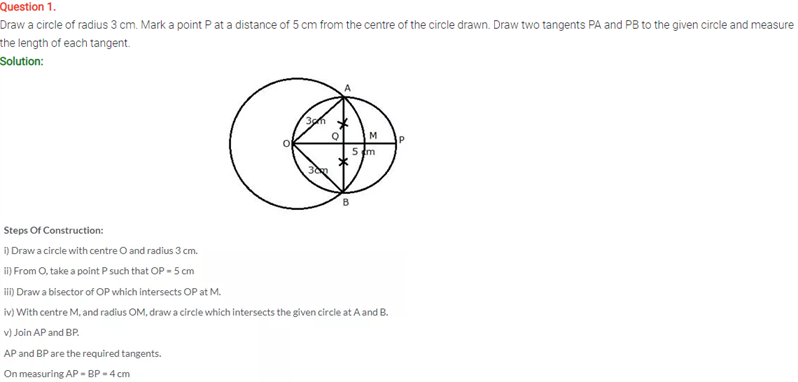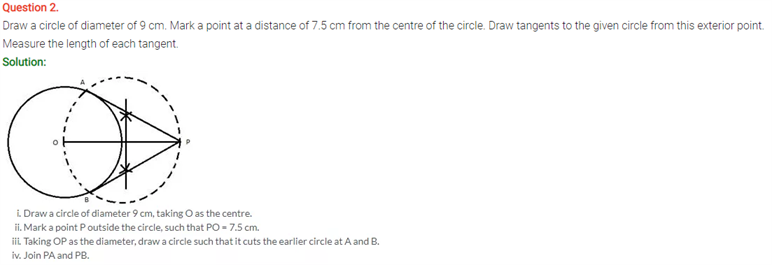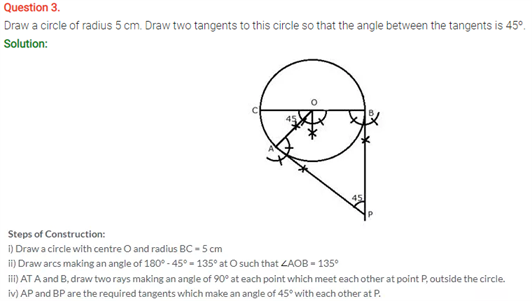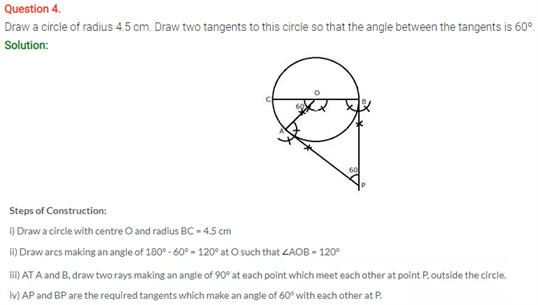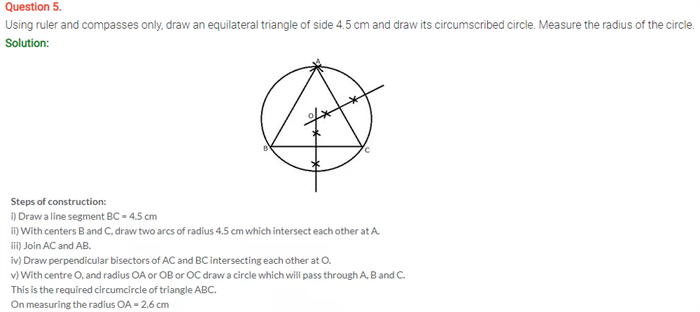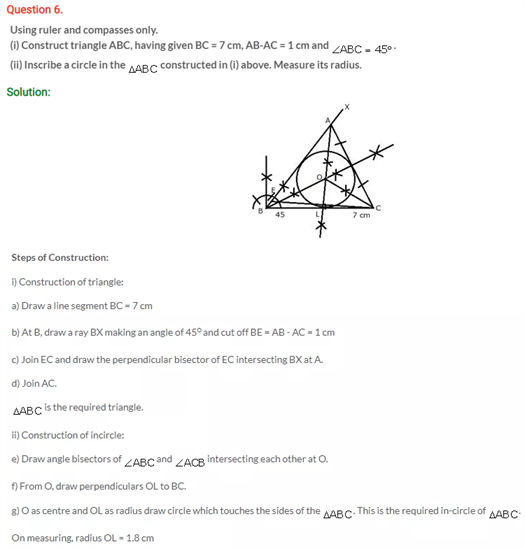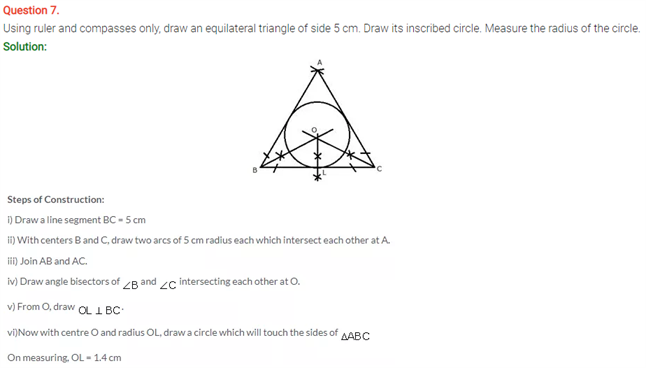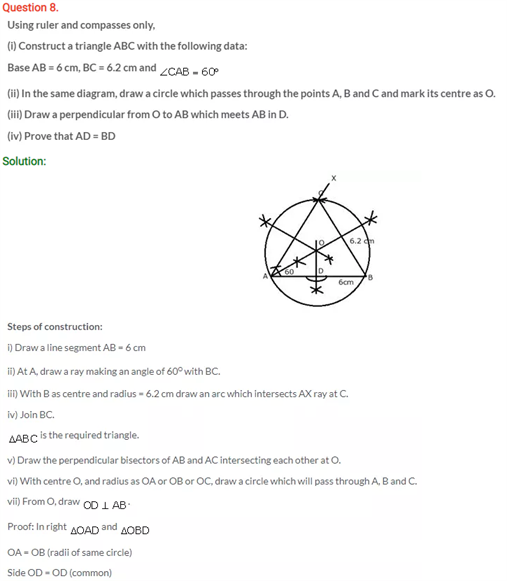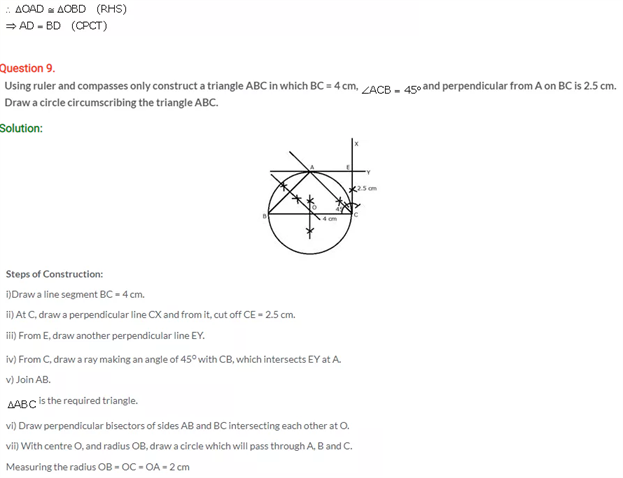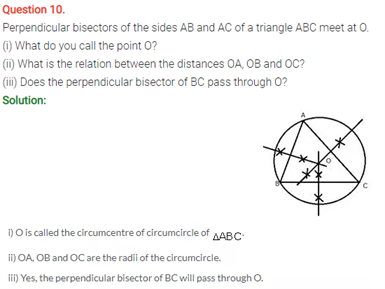# Selina Concise Maths Solution for ICSE Class 10 Chapter 19 Constructions (Circles)

## Selina Class 10 Maths Solutions for Chapter 19

In a circle’s construction, solving the solutions on how to draw the circle properties from the ICSE Selina Solutions For Class 10 Maths will give you clarity on this chapter. Drawing a chord of a line segment connecting two points in a circle, and making tangent such as a line passing a circle and touching it at only one point. Getting to know to carve out a geometrical figure with the given measurements and keeping in mind the basic properties such as a line which touches a circle or ellipse at just one point is called a tangent and the radius to the point of tangency is always perpendicular to the tangent line. These questions below belong to the above concepts.

Check out the Class 10 Selina Maths ICSE Solutions Chapter 19 Constructions below: